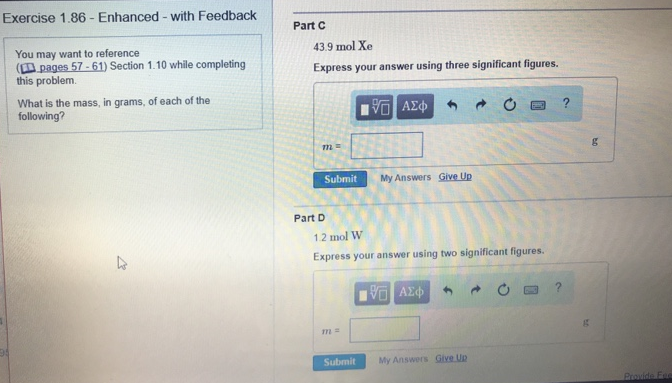# Problem: What is the mass, in grams, of each of the following?Part C43.9 mol XeExpress your answer using three significant figures.Part D1.2 mol WExpress your answer using three significant figures.

###### FREE Expert Solution
87% (494 ratings)###### Problem Details

What is the mass, in grams, of each of the following?

Part C

43.9 mol Xe

Part D

1.2 mol W